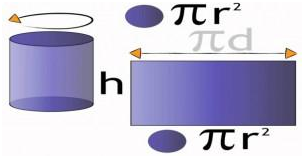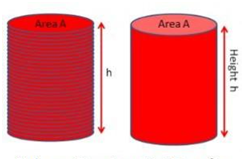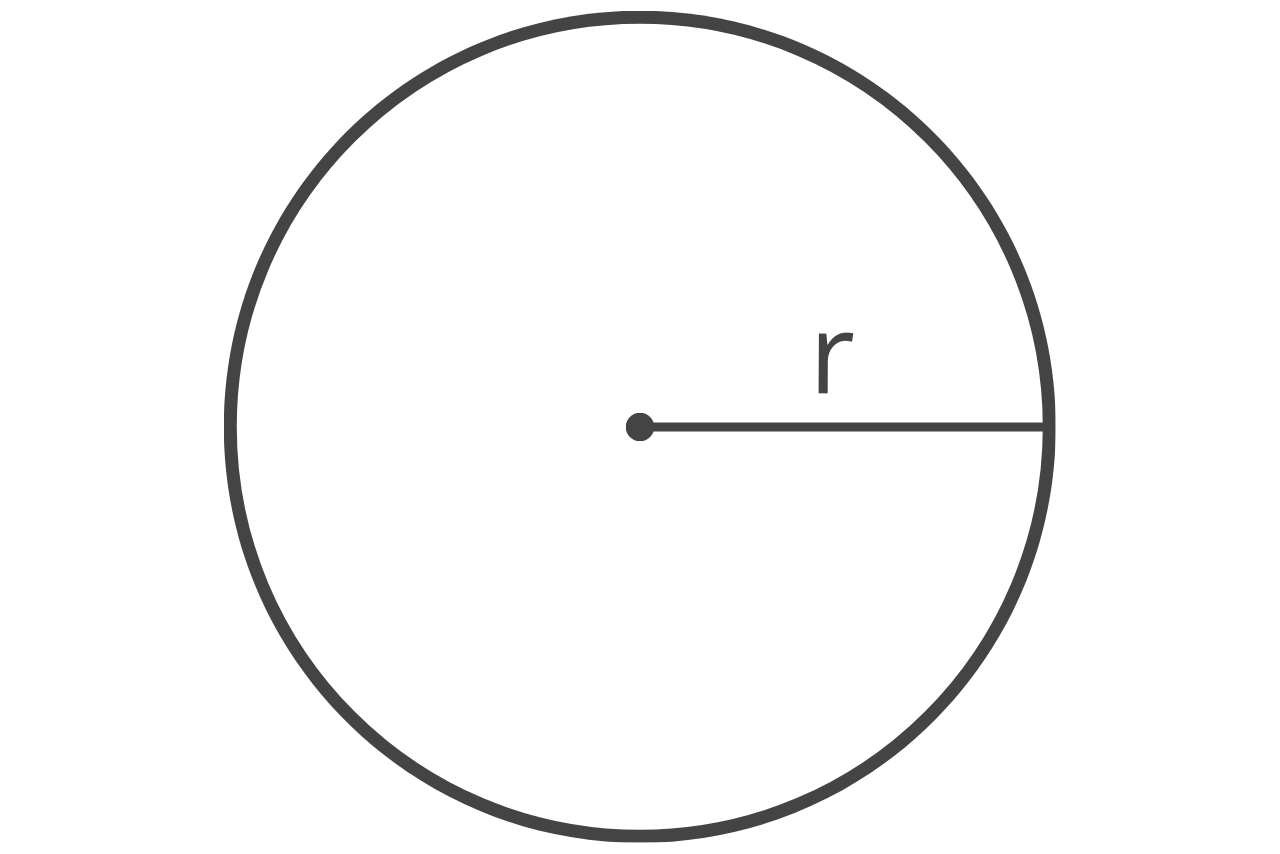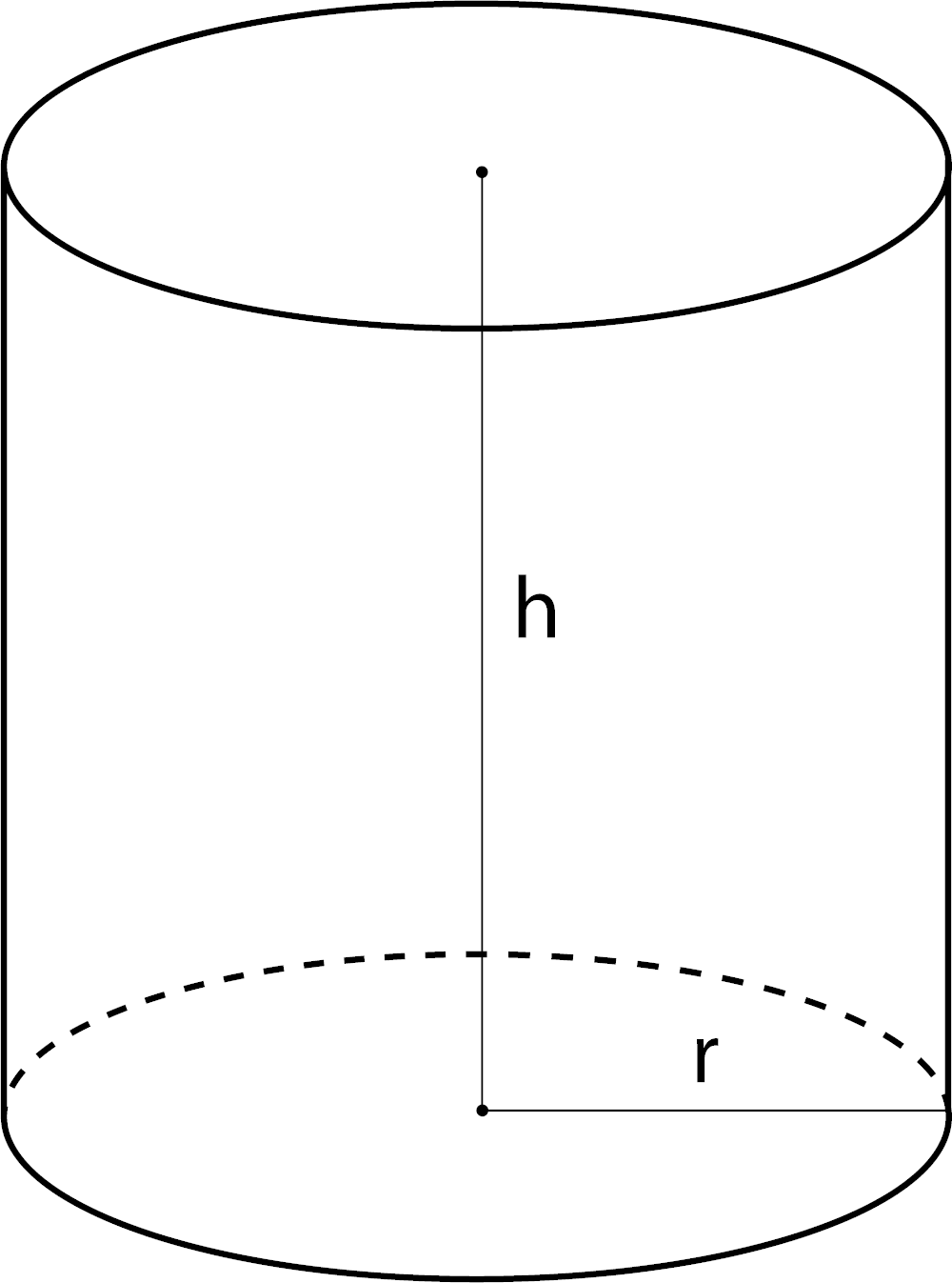# How to calculate volume of a cylinderA cylinder is a 3D structure that has two circular faces which are joined by a curved surface. A cylinder can be viewed as stacks of circular discs which are placed on one another. The total surface area of a cylinder can be calculated by summing up the areas of all its faces.  Volume of a cylinder is its capacity. It is the amount of space occupied by it. Mathematically, we can express it as:$$Volume\; of\; a\; cylinder =\pi r^{2}h$$

Where r refers to the base radius and h is the height of the cylinder.

Derivation:

As we mentioned, a cylinder is nothing but a set of circular discs stacked one upon the other. So if we compute the space occupied by each of these discs and add them up, what we get is the volume of the cylinder.

Let us say that the circular discs are stacked up to the height of ‘h’. Now, the volume of cylinder will be the product of the base area of the discs and the height ‘h’.Volume of the cylinder = Area of the circular base × Height

Area of the circular base = $$\pi r^{2}$$Height = h

Thus, volume of a cylinder of height ‘h’ and base radius ‘r’ can be given as $$\pi r^{2}\times h$$

$$Volume\; of\; a\; cylinder =\pi r^{2}h$$

Volume of a Cylinder Formula in Real Life:

In our daily routine, we come across different types of situations where we have to handle cylindrical objects. The volume of cylinder formula is useful in calculating the capacity or volume of such cylindrical objects. This calculation can also help in designing cylindrical containers of larger or smaller volume as per the need. For example, the designing of water tanks, perfume bottles, cylindrical containers, cylindrical flasks you use in chemistry labs, etc.  You can easily find out the volume of a cylinder if you have the measurements of its height and radius.

Solved Examples:

Related text  How to get views on youtube

Question 1: The volume and height of a cylindrical container are 440 m3 and 35m respectively. Calculate its radius of the base. (Take $$\pi =\frac{22}{7}$$)

Solution: Given,

Volume of the cylindrical container = 440 m³

Height of the cylindrical container = 35 m

$$Volume\; of\; a\; cylinder =\pi r^{2}h$$

Or 440 m³= $$\pi r^{2}h$$

=>$$r^{2}=\frac{440}{\pi h}=\frac{440\times 7}{22\times 35}$$

=> r² = 4m

=> r = 2 m

The radius of the base of the cylindrical container is 2 m.

Question 2: How many litres of water can a cylindrical water tank with base radius 20 cm and height 28 cm hold?

Solution: Given,

Base radius of the cylindrical water tank, r = 20 cm

Height of the cylindrical water tank, h = 28 cm

Volume of the cylindrical water tank = $$\pi r^{2}h$$

Volume of the cylindrical water tank = $$\frac{22}{7}\times 20^{2}\times 28=35200\; cm^{3}$$

1 cubic centimeter = 0.001 litre =$$1\times 10^{-3}$$ litre

∴ 35200 cubic centimeter = $$35200\times 10^{-3}$$ = 35.2 litres

The cylindrical water tank can hold 35.2 litres of water.

To solve more problems on the topic, download Byju’s -The Learning App.

This calculator is perfect for finding the cubic volume of a cylinder, given the radius or the diameter and the height. This is useful for determine cubic yardage for concrete projects, filling sonotubes, posts, or footings, building and construction projects, or landscaping projects. This is also helpful for anyone trying to find the volume of a cylinder. Estimate the cost for materials to fill the cylinder by entering a price per cubic yard.

## How to Find the Cubic Yardage of a Cylinder

The cubic volume of a cylinder is found by multiplying the radius times the radius times pi times the height.

• Find the radius and height. If the diameter is known then divide the diameter by 2 to get the radius. The radius and height should be in yards, if you have other measurements convert to yards.
• Convert inches to yards, convert feet to yards, or convert meters to yards
• Multiply the radius of the cylinder times the radius, eg. r2
• Multiply times π to get the area of the circle
• Multiply times the height of the cylinder to get the volume
• Multiply the cubic yardage by the price per cubic yard to get the cost of materials, if required
Related text  How to paint a galaxy

area = π × (diameter ÷ 2)2area = π × r2

π = 3.14159265359volume = π × (diameter ÷ 2)2 × heightvolume = π × r2 × h

π = 3.14159265359Our concrete calculator can also help estimate concrete material for a sonotube or footing.